All submissions of the EM system will be redirected to Online Manuscript Submission System. Authors are requested to submit articles directly to Online Manuscript Submission System of respective journal.Sharafat Ali*

Department of Mathematics, Aga Khan University, Pakistan, Email: [email protected]

*Correspondence: Sharafat Ali, Professor, Department of Mathematics, Aga Khan University, Pakistan, Email: [email protected]

Received: 01-Sep-2021 Accepted Date: Sep 15, 2021; Published: 22-Oct-2021

Citation: Ali S. Sine gordon expansion method. J Pur Appl Math. 2021; 5(5):61:66.

This open-access article is distributed under the terms of the Creative Commons Attribution Non-Commercial License (CC BY-NC) (http://creativecommons.org/licenses/by-nc/4.0/), which permits reuse, distribution and reproduction of the article, provided that the original work is properly cited and the reuse is restricted to noncommercial purposes. For commercial reuse, contact [email protected]

## Abstract

In the field of applied mathematics, fractional calculus is used to contract with derivative as well as the integration of any power. Different definitions of the fractional derivative have been introduced in the literature. For example, these are some important definitions of fractional derivatives, Riemann-Liouville derivative, Caputo derivative and conformable derivative. Recently the generalization of the conformable derivative has been given as M-fractional conformable. Fractional differential equations (FDEs) “equations involving fractional derivatives” are employed invarious areas of science and engineering and others have widely been interested. That’s why they have gained many attractions from many researchers. To acquisition, the analytical solutions of the FPDEs are a conspicuous look of scientific research.

### Keywords

Fractional Derivative; Riemann-Liouville derivative; Conformable derivative

### Introduction

Consequently, numerous scholars have developed some persuasive methods to acquired approximate and exact solutions for these types of FPDEs. In this investigation, the truncated M-fractional conformable (STO) and (3 + 1)-dimensional KdV-ZK equations are considered. The novel method: (m + G′/G )-expansion method are utilized to extract the exact solutions of the aforesaid model equations. Different definitions of fractional derivatives have appeared in the literature. For example, Reimann- Liouville , Caputo derivative and conformable derivative. Despite these two most recent definitions are reported as M-fractional conformable and beta derivatives. Many powerful techniques have been reported in the literature for finding exact solutions; see for example [2-9]. Fortunately, it is possible to establish a traveling wave transformation for a fractional order PDE which can convert it to a nonlinear ordinary differential equation (NODE) that can be easily solved by using a variety of different methods. There are many distinct techniques have been applied to gain the exact solutons of (STO) and (3 + 1)-dimensional KdV-ZK equations like : F-expansion method and Improved - expansion methods are used to obtain the bright, dark 1-soliton and other soliton solutions , new extended direct algebraic technique is implemented to gain the number of new type of solutions of the conformable fractional (STO) equation , dark and bright optical solutions gained by variable Coefficient method , dispersive exact wave solutions are observed by modified simplest equation method , different optical solutions of the (STO) equation are achieved by applying the extended trial method , extended sinh-Gordon equation expansion scheme has utilized to Obtain different types of optical solutions of STO equation , extended auxiliary equation scheme is applied to gain the dispersive optical wave solutions of time-fractional (SH) equation along power law non-linearity as well as Kerr Law non-linearity , undetermined coefficient method is implemented to gain the distinct kinds of dispersive exact solutions in the presence of several perturbation terms are achieved , with the use of tanh- coth integration algorithm dispersive solutions in optical nanofibers are obtained with constraint conditions , by using the Sine-Cosine function method, different exact solutions are obtained , Sech, Tanh and Csch function techniques are utilized to gain the optical solutions of (STO) equation along Kerr law non-linearity .

There are many applications of the Sine-Gordon expansion method and (m + G′/G) -expansion method. For instance, with the use of Sine Gordon-expansion scheme,distinct kinds of solutions of the non-linear Time-fractional Biological Population equation and the Cahn- Hilliard model have been obtained in , hyperbolic and trigonometric Functions solutions to the non-linear reaction diffusion equation have achieved  etc. Similarly, (m + G′/G)-expansion method has been utilized to solve the Pochhammer- Chree equations for bell-shaped, kink-shaped and periodic type solutions of with the help of this method , discrete and periodic type solutions of the Ablowitz-Ladik lattice system are found  etc.

To find the exact solutions of integrable partial differential equations is the most interesting topic. Therefore, we will solve two integrable model equations namely space-time fractional Sharma Tasso-Olever (STO) and space- time fractional (3+1)-dimensional KdV-ZK equations for a variety of solutions with a novel derivative operator by employing (m + G′/G)- expansion method: Abundant M-Fractional Exact Solutions for STO and (3+1)-Dimensional KdV-ZK Equations via (m + G′/G)-Expansion Method.

### Sine Gordon Expansion Method

Consider the sine-Gordon equation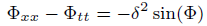(1)

Where Φ = Φ(x, t) and δ is a non-zero real number using the wave transform(2)

On equation (1) and simplifying the results, we get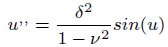(3)

Where u = u(ξ) ,ξ and ν are the amplitude and speed of travelling wave. Integrating Eq.( 3) and simplifying it, one can get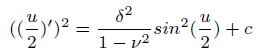(4)

Where c is the constant of integration. Suppose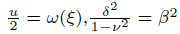and putting them into eqn.(4), the result is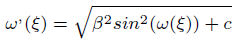(5)

Taking c=0 and 1, the solution of Eq(5),becomes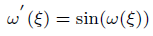(6)

Using the separation method, Eq.(6) possesses the solution as follows: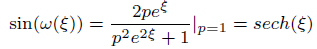(7)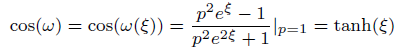(8)

Where P is the constant of integral. These two significant solutions achieve the definition of the SGEM to get the solution of the NPDE of the form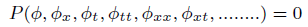(9)

Now, we consider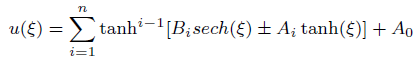(10)

Now, due to equation 7 and equation 8, equation 10 can be rewritten as follows: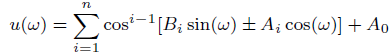(11)

The value of n is obtained by balancing the highest power of nonlinear term and the highest derivative appearing in the transformed NODE.

### Applications

The Space-Time Fractional STO Equation

Consider the space-time fractional order STO equation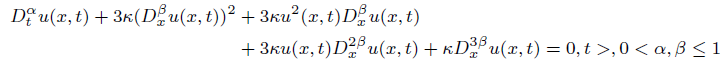(12)

Using the transformation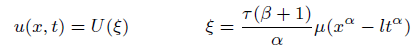(13)

12 can be changed into an ODE equation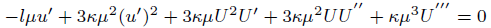(14)

Where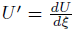We get M = 1 as a result of balancing terms U 2U′ and U’’’ in 14 the solution can therefore, expressed as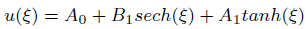(15)

Where A0, A1, B1 are constant to be computed.

Description of Sine-Gordon Expansion method , will yield the following system of algebraic equations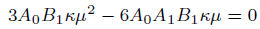(16)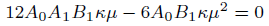(17)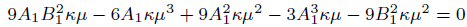(18)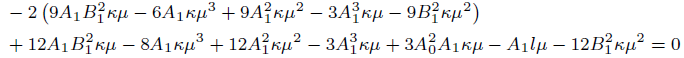(19)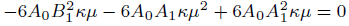(20)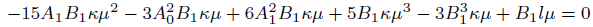(21)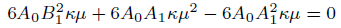(22)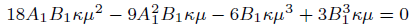(23)

case 01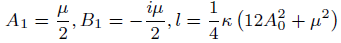(24)

case 02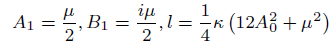(25)

case 03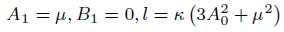(26)

case 04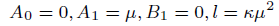(27)

case 05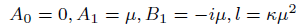(28)

case 06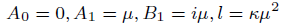(29)

case 07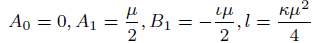(30)

case 08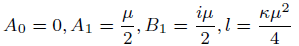(31)

case 09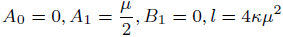(32)

Solution1 (corresponding to case 01) (Figure 1)

Figure 1: Solution of (33) κ = 0.6, μ = 0.5, β = 2, A0 = 0.05.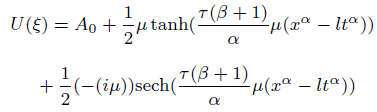(33)

Solution2 (corresponding to case 02)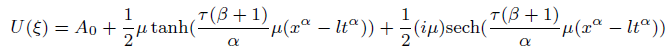(34)

Solution3 (corresponding to case 03)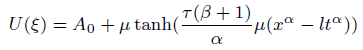(35)

Solution4 (corresponding to case 04) (Figures 2-10)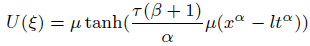(36)

Figure 2: Solution of (33) κ = 0.6, μ = 0.5, β = 2, A0 = 0.05.

Figure 3: Solution of (33) κ = 0.6, μ = 0.5, β = 2, A0 = 0.05.

Figure 4: Solution of (33) κ = 0.6, μ = 0.5, β = 2, A0 = 0.05.

Figure 5: Solution of (33) κ = 0.6, μ = 0.5, β = 2, A0 = 0.05

Figure 6: Solution of (34) when κ = 0.6, μ = 0.5, β = 2, A0 = 0.05.

Figure 7: Solution of (34) when κ = 0.6, μ = 0.5, β = 2, A0 = 0.05.

Figure 8: Solution of (34) when κ = 0.6, μ = 0.5, β = 2, A0 = 0.05.

Figure 9: Solution of (34) when κ = 0.6, μ = 0.5, β = 2, A0 = 0.05.

Figure 10: Solution of (41) κ = 0.6, μ = 0.5, β = 2, A0 = 0.05.

Solution5 (corresponding to case 05)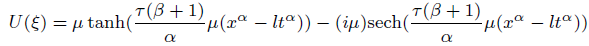(37)

Solution6 (corresponding to case 06)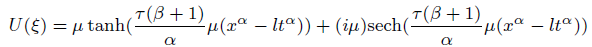(38)

Solution7 (corresponding to case 07)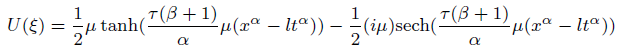(39)

Solution8 (corresponding to case 08)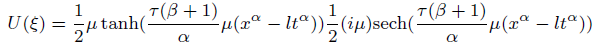(40)

Solution9 (corresponding to case 09) (Figures 11-14)

Figure 11: Solution of (41) κ = 0.6, μ = 0.5, β = 2, A0 = 0.05.

Figure 12: Solution of (41) κ = 0.6, μ = 0.5, β = 2, A0 = 0.05.

Figure 13: Solution of (41) κ = 0.6, μ = 0.5, β = 2, A0 = 0.05.

Figure 14: Solution of (41) κ = 0.6, μ = 0.5, β = 2, A0 = 0.05.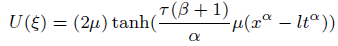(41)

Space-Time Fractional Kdv-ZK Equation by Sine-Gordon Expansion Method

Consider the space-time fractional Kdv-ZK equation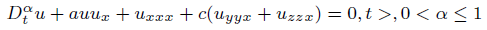(42)

And the transformation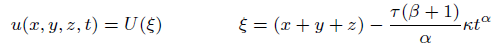(43)

Where κ is nonzero constant, which on substituting in 42 give us the following ODE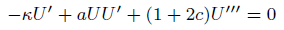(44)

The trial solutions of (44) can be expressed as

U(𝜉) = tanh(𝜉) (A2 tanh(𝜉) + B2sech(𝜉))+A1 tanh(𝜉)+A0+B1sech(𝜉)UsingEqs(??)intoEqs(44)theAlgebraicequationscanbewrittenas -aA0B1 aA21 +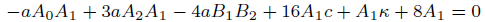(45)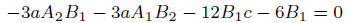(46)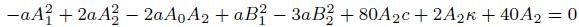(47)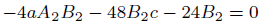(48)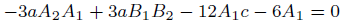(49)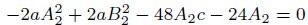(50)

case 01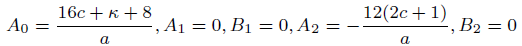(51)

case 02(52)

case 03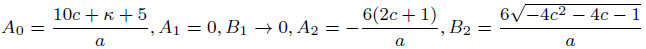(53)

Solution corresponding to case 01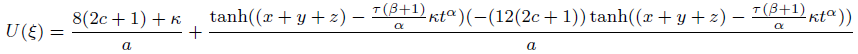(54)

Solution corresponding to case 02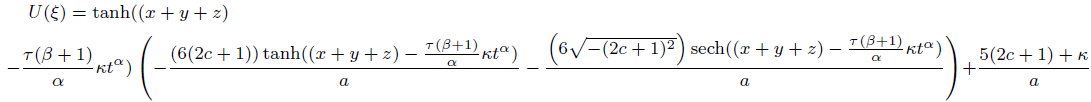(55)

Solution corresponding to case 03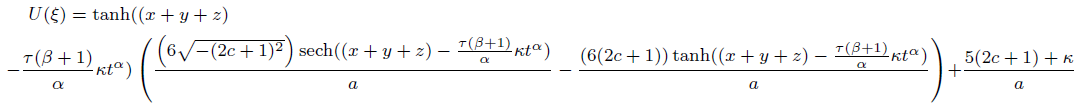(56)

### Conclusion

By applying a complex wave transformation, we have be successful to obtain the solitons of the metamaterials with anti-cubic law of non-linearity. We have discussed the optical soliton solutions, along with some constrained conditions on parameters, of the metamaterials with anti-cubic law of nonlinearity via the modi_ed tanh expansion method. Many new optical solitary and periodic solitary wave solutions have been retrieved with the above afore mentioned integration scheme. These solutions are thus very encouraging to carry out future studies in this area.

## Recommended Conferences

### 7th Annual Meet on Advances in Physics, Mathematics and Applied Science

Budapest, HungaryTop